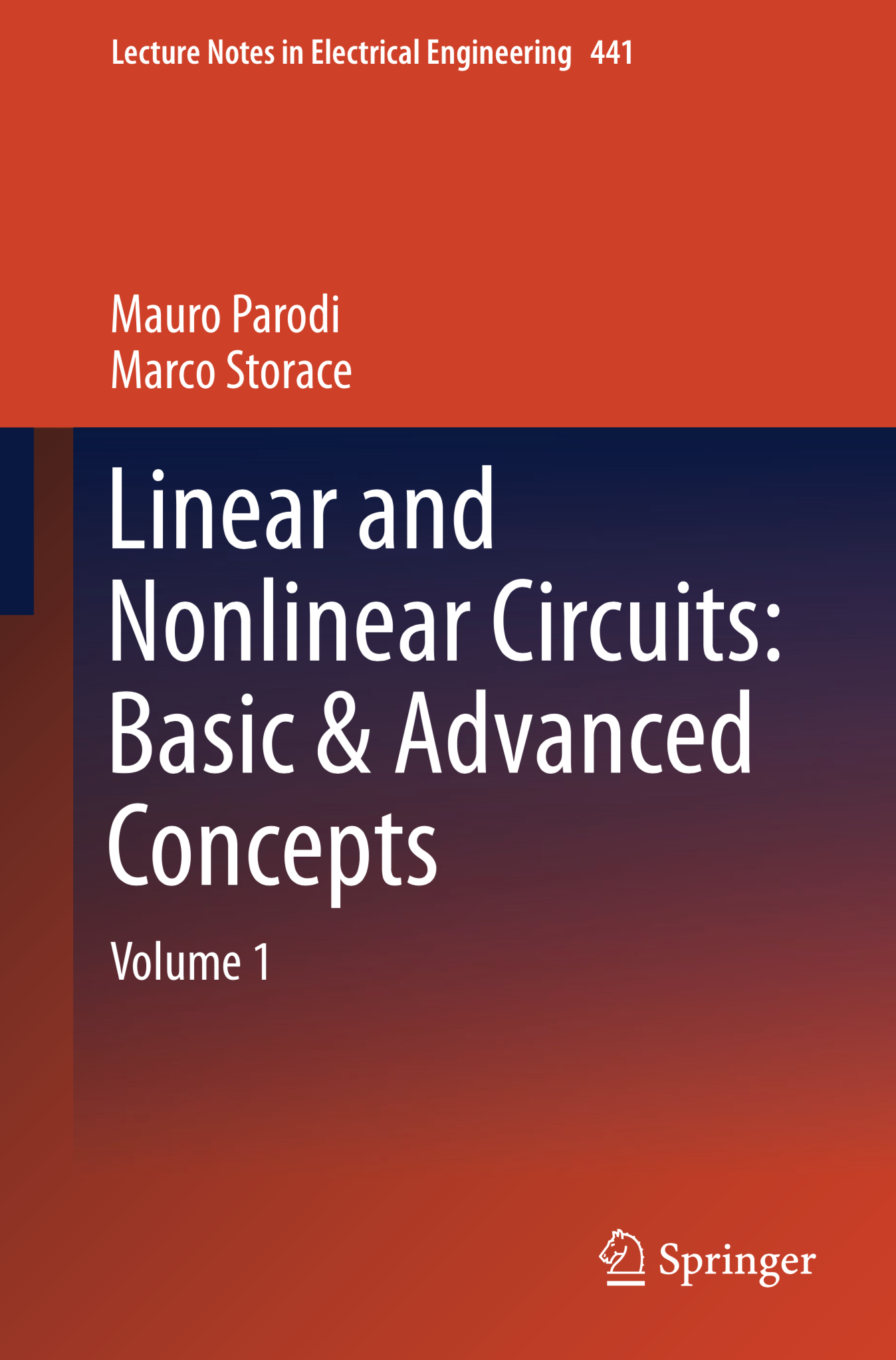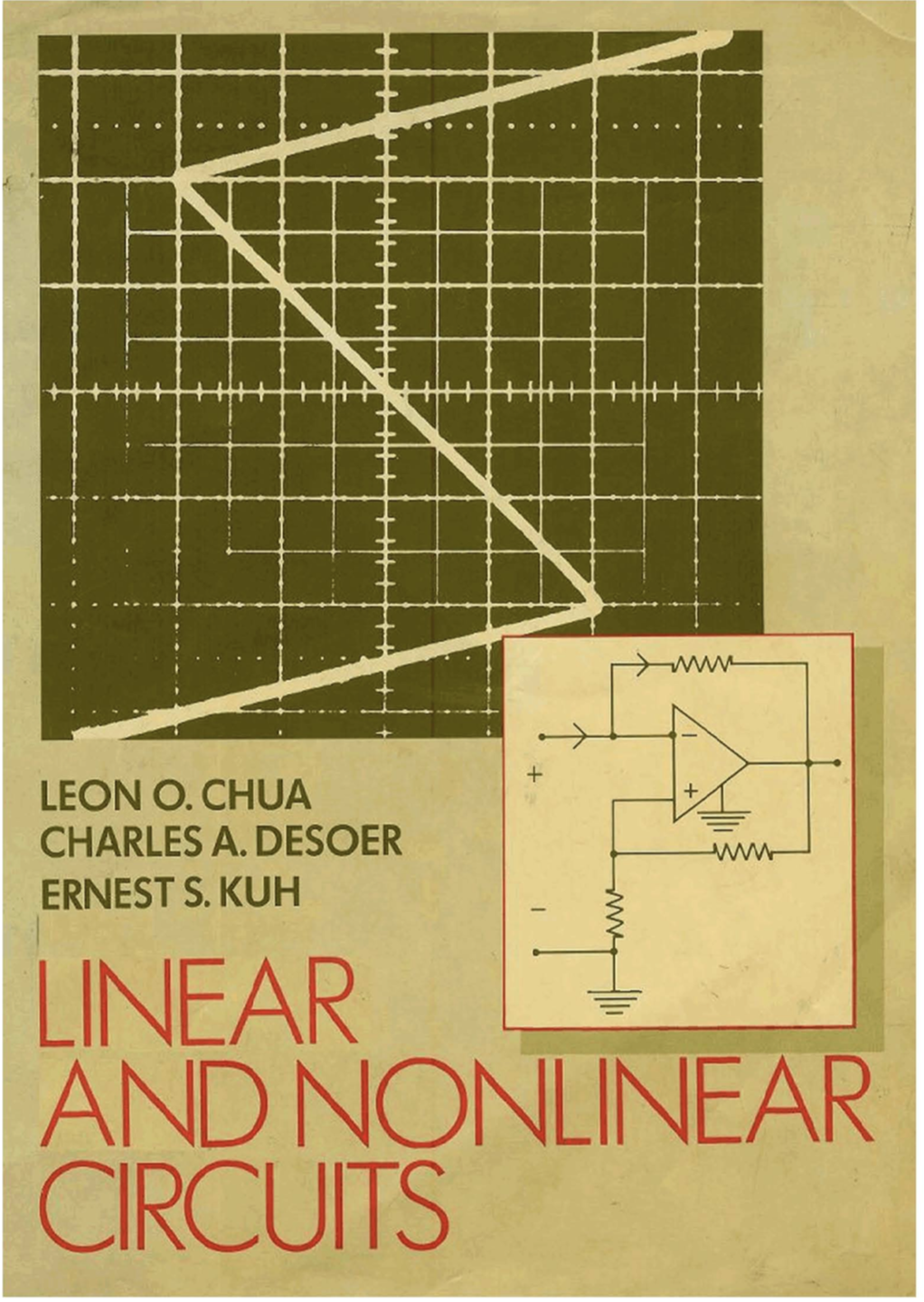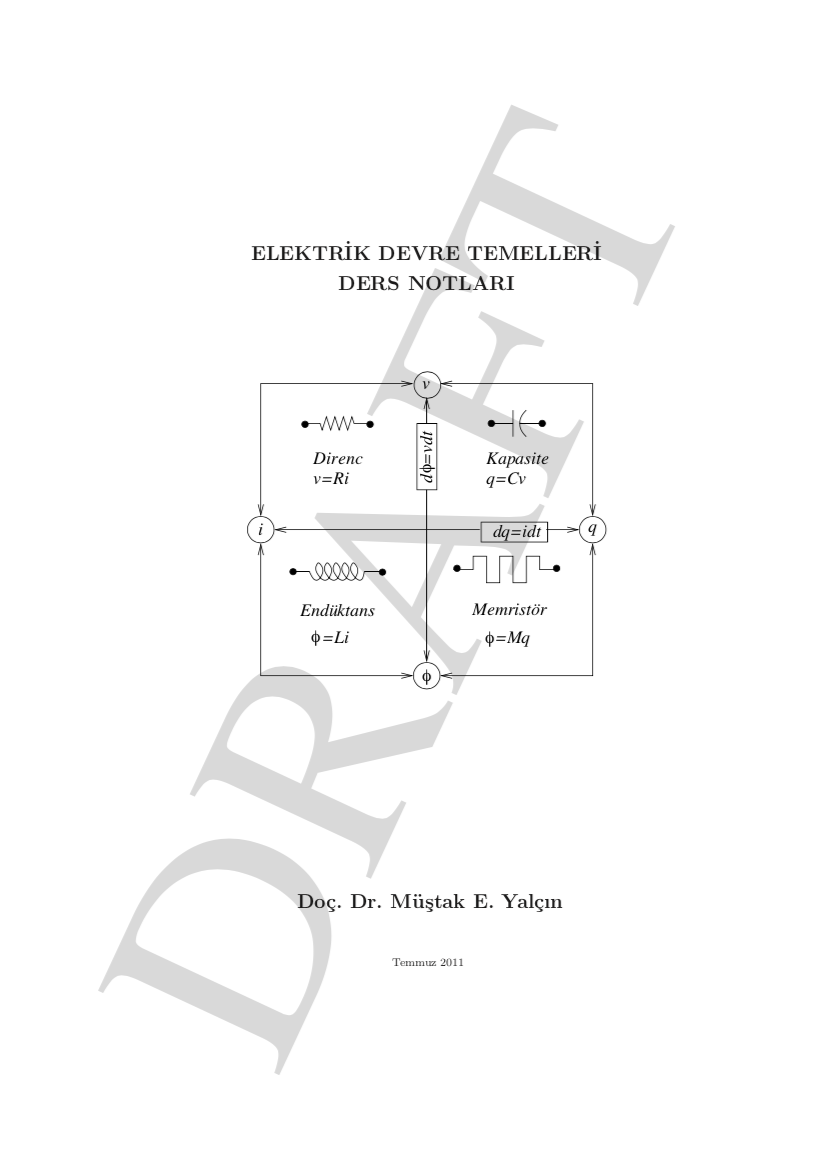## EHB211E Basics of Electrical Circuits

e-mail: mustak.yalcin [at] itu.edu.tr

### Description:

Electric circuits, Models and Circuits elements. Kirchhoff’s laws: Kirchhoff’s voltage law and Kirchhoff’s current law. Graph theory, element graph: Branch currents, branch voltages, Graph matrices. Tellegen Theorem and Conservation of energy. Two terminal elements: resistor, capacitor and inductor. Independent sources, dependent sources. Three terminal elements: Gyrator, transistor, transformer. Nonlinear elements Linearized models. Node voltage method and mesh current method for resistive circuits. Thevenin and Norton equivalent circuits. RLC circuits: First order and second order circuits. State equation and state variables for linear time invariant circuits. Solution of second order state equations.

Course Catalogue Form: EHB211E

### Textbooks>